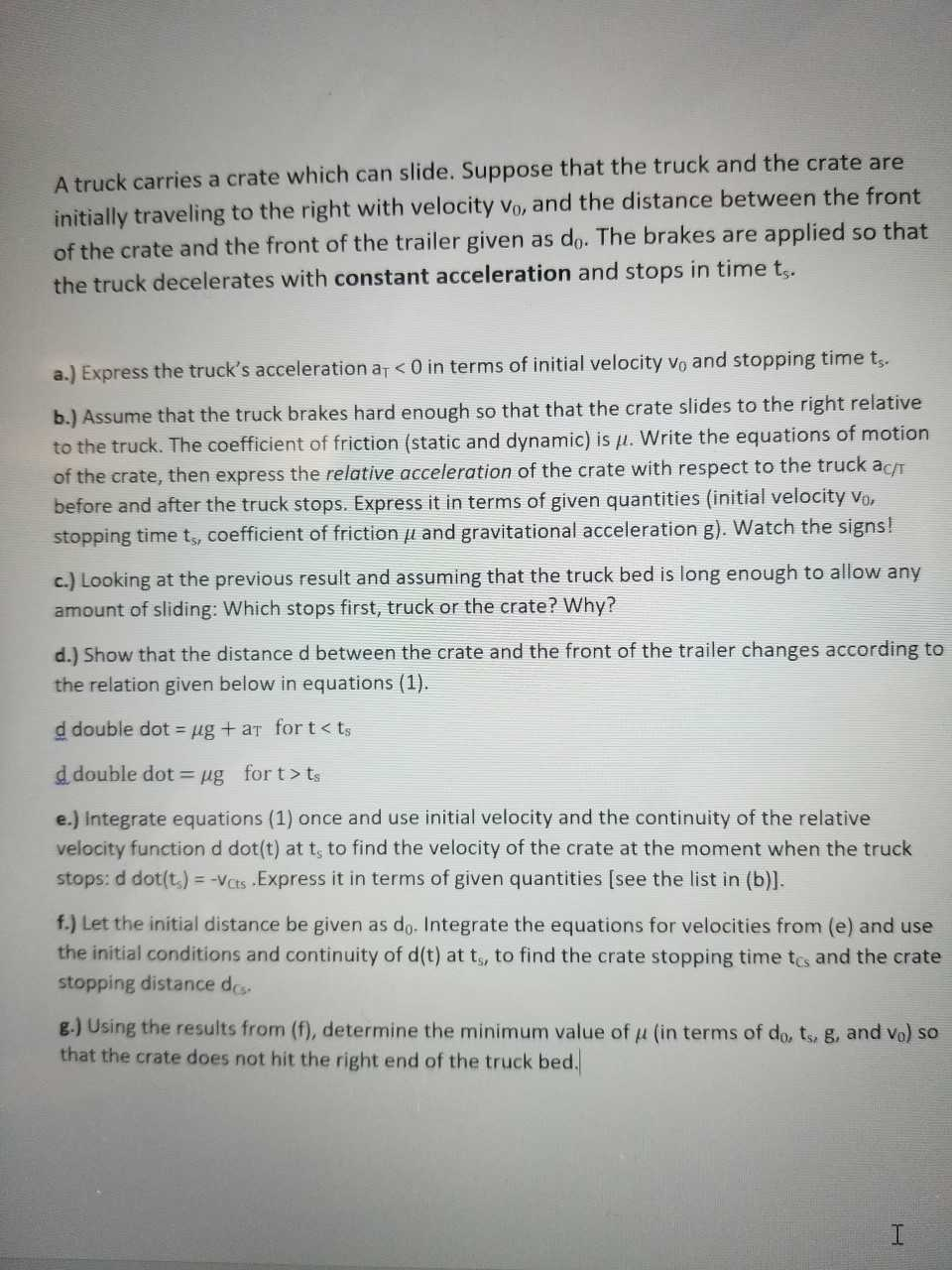# A truck carries a crate which can slide. Suppose that the truck and the crate are initially traveling to the right with velocity Vo, and the distance between the front of the crate and the front of the trailer given as do. The brakes are applied so that the truck decelerates with constant acceleration and stops in time t a.) Express the truck's acceleration ar <0 in terms of initial velocity vo and stopping time t.. b.) Assume that the truck brakes hard enough so that that the crate slides to the right relative to the truck. The coefficient of friction (static and dynamic) is μ. write the equations of motion of the crate, then express the relative acceleration of the crate with respect to the truck acr before and after the truck stops. Express it in terms of given quantities (initial velocity vo stopping time t, coefficient of friction μ and gravitational acceleration g). watch the signs! c.) Looking at the previous result and assuming that the truck bed is long enough to allow any amount of sliding: Which stops first, truck or the crate? Why? d.) Show that the distance d between the crate and the front of the trailer changes according to the relation given below in equations (1). d double dot = μg + aT for t < ts d double dot-lug for t > ts e.) Integrate equations (1) once and use initial velocity and the continuity of the relative velocity function d dot(t) at t, to find the velocity of the crate at the moment when the truck stops: d dot(t,)--Vats.Express it in terms of given quantities [see the list in (b)]. f.) Let the initial distance be given as do. Integrate the equations for velocities from (e) and use the initial conditions and continuity of d(t) at ts, to find the crate stopping time tcs and the crate stopping distance des g.) Using the results from (f), determine the minimum value of μ (in terms of do, ts, g, and v ) so that the crate does not hit the right end of the truck bed.

Question

Please refer to the attached picturs of the problem statement. I have solved a, b, c, and g using kinematics and Newton's Second Law. I'm not sure how to answer d, e and f however.  My answers are:

a) aT=v0/ts

b) ac/t=mu(g)+v0/ts

c)truck stops before crate

d) unsure

e) unsure

f) unsure

g) mu=[(aTts)2]/[2d0-2v0ts-aTts2]

mu=[aTv02]/[g(2d0aT-3v02)]help_outlineImage TranscriptioncloseA truck carries a crate which can slide. Suppose that the truck and the crate are initially traveling to the right with velocity Vo, and the distance between the front of the crate and the front of the trailer given as do. The brakes are applied so that the truck decelerates with constant acceleration and stops in time t a.) Express the truck's acceleration ar <0 in terms of initial velocity vo and stopping time t.. b.) Assume that the truck brakes hard enough so that that the crate slides to the right relative to the truck. The coefficient of friction (static and dynamic) is μ. write the equations of motion of the crate, then express the relative acceleration of the crate with respect to the truck acr before and after the truck stops. Express it in terms of given quantities (initial velocity vo stopping time t, coefficient of friction μ and gravitational acceleration g). watch the signs! c.) Looking at the previous result and assuming that the truck bed is long enough to allow any amount of sliding: Which stops first, truck or the crate? Why? d.) Show that the distance d between the crate and the front of the trailer changes according to the relation given below in equations (1). d double dot = μg + aT for t < ts d double dot-lug for t > ts e.) Integrate equations (1) once and use initial velocity and the continuity of the relative velocity function d dot(t) at t, to find the velocity of the crate at the moment when the truck stops: d dot(t,)--Vats.Express it in terms of given quantities [see the list in (b)]. f.) Let the initial distance be given as do. Integrate the equations for velocities from (e) and use the initial conditions and continuity of d(t) at ts, to find the crate stopping time tcs and the crate stopping distance des g.) Using the results from (f), determine the minimum value of μ (in terms of do, ts, g, and v ) so that the crate does not hit the right end of the truck bed. fullscreen